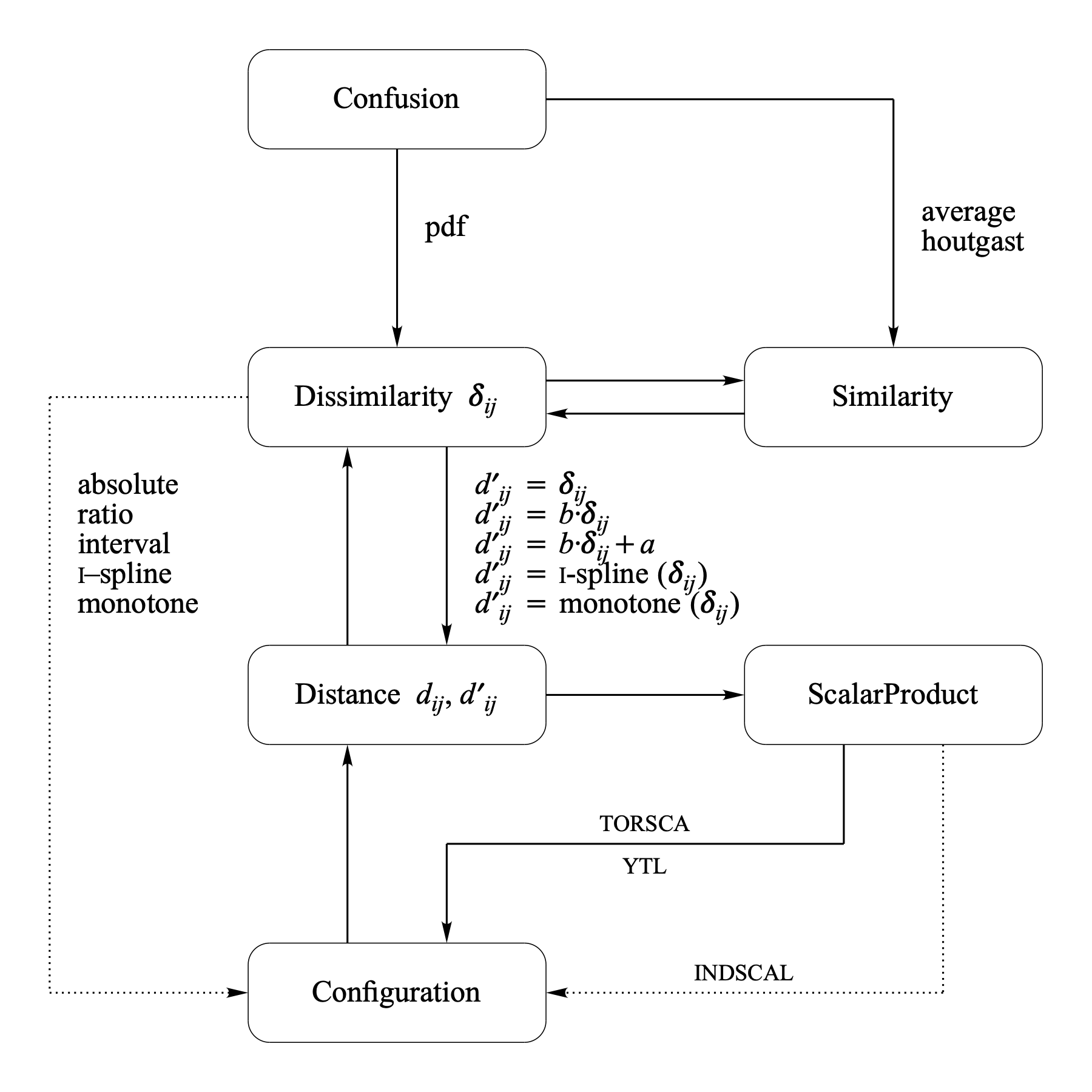MDS models

Multidimensional scaling (MDS) models are defined by specifying how given Dissimilarity data, δij, are mapped into distances of an m-dimensional MDS Configuration X. The mapping is specified by a representation function, f : δijdij(X), which specifies how dissimilarities should be related to the distances. The MDS analysis tries to find the configuration (in a given dimensionality) whose distances satisfy f as closely as possible. This closeness is quantified by a badness-of-fit measure which is often called stress.

### Representation functions

In the application of MDS we try to find a configuration X such that the following relations are satisfied as well as possible:

 f(δij) ≈ dij(X)

The numbers that result from applying f on δij are sometimes called disparities dij. In most applications of MDS, besides the configuration X, also the function f is not completely specified, i.e., the exact parameters of f are unknown and must also be estimated during the analysis. If no particular f can be derived from a theoretical model, one often restricts f to a particular class of functions on the basis of the scale level of the dissimilarity data. If the disparities are related to the proximities by a specific parametric function we speak of metric MDS otherwise we speak of ordinal or non-metric MDS.

•  absolute mds: dij = δij
• No parameters need to be estimated.
•  ratio mds: dij = b · δij,
• where the value of b can be estimated by a linear regression of dij on δij.
•  interval mds: dij = a + b · δij,
• where the values of a and b can be estimated by a linear regression of dij on δij.
•  i-spline mds: dij = i-spline(δij),
• where i-spline(·) is a smooth monotonically increasing spline curve. The conceptual idea is that it is not possible to map all dissimilarities into disparities by one simple function.
•  monotone mds: dij = monotoneij),
• where monotone(·) is restricted to be a monotonic function that preserves the order of the dissimilarities:
 if δij < δkl, then dij(X) < dkl(X)
If δij = δkl and no particular constraint is involved for dij(X) and dkl(X) this is referred to as the primary approach to ties. The secondary approach to ties requires that if δij = δkl, then also dij(X) = dkl(X).

More information on all aspects of multidimensional scaling can be found in: Borg & Groenen (1997) and Ramsay (1988).

The most important object types used in Praat for MDS and the conversions between these types are shown in the following figure.